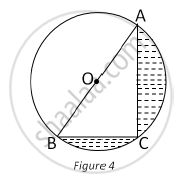# In Fig. 4, O is the centre of a circle such that diameter AB = 13 cm and AC = 12 cm. BC is joined. Find the area of the shaded region. - Mathematics

In Fig. 4, O is the centre of a circle such that diameter AB = 13 cm and AC = 12 cm. BC is joined. Find the area of the shaded region. (Take π = 3.14)#### Solution

Diameter, AB = 13 cm

∴ Radius of the circle, r=13/2=6.5 cm

∠ACB is the angle in the semi-circle.

∴ ∠ACB = 90°

Now, in ACB, using Pythagoras theorem, we have

AB2=AC2= BC2

∴ (13)2=(12)2+(BC)2

∴ (BC)2 =(13)2-(12)2=169-144=25

∴ BC = sqrt25=5cm

Now, area of shaded region= Area of semi-circle- Are of ΔACB

=1/2pir^2 -1/2xxBCxxAC

=1/2xx3.14xx(6.5)^2-1/2xx5xx12

= 66.33-30

= 36.33 cm2

Thus, the area of the shaded region is 36.33 cm2 .

Concept: Circumference of a Circle
Is there an error in this question or solution?
2015-2016 (March) All India Set 1

Share